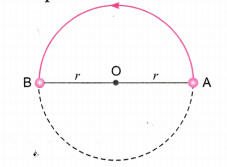# A particle is moving in a circular path of radius r. What would be the displacement after half a circle?

A particle is moving in a circular path of radius r. What would be the displacement after half a circle?